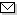Language:   Search:   Contact# Search and Browse the MSC 2000

Search:
Please note that in the second half of 2009, MSC2010 is deployed in the production of ZMATH and will become official standard in January 2010.

Enter a query and click »Search«...

57-XX Manifolds and cell complexes {For complex manifolds, see 32Qxx} → ZMATH
57Nxx Topological manifolds → ZMATH
57N05 Topology of $E^2$, $2$-manifolds → ZMATH
57N10 Topology of general $3$-manifolds [See also 57Mxx] → ZMATH
57N12 Topology of $E^3$ and $S^3$ [See also 57M40] → ZMATH
57N13 Topology of $E^4$, $4$-manifolds [See also 14Jxx, 32Jxx] → ZMATH
57N15 Topology of $E^n$, $n$-manifolds ($4 < n < \infty$) → ZMATH
57N17 Topology of topological vector spaces → ZMATH
57N30 Engulfing → ZMATH
57N35 Embeddings and immersions → ZMATH
57N37 Isotopy and pseudo-isotopy → ZMATH
57N40 Neighborhoods of submanifolds → ZMATH
57N45 Flatness and tameness → ZMATH
57N50 $S^{n-1}\subset E^n$, Schoenflies problem → ZMATHAbel prize 2010I. M. Gelfand 1913-2009MSC2010